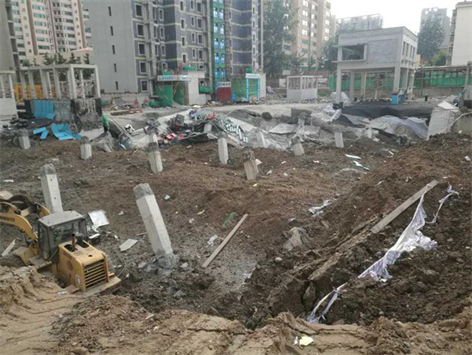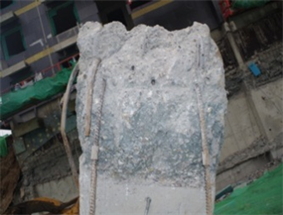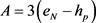﻿ 混凝土板柱节点弯曲裂缝截面冲切承载力验算方法研究

# 混凝土板柱节点弯曲裂缝截面冲切承载力验算方法研究Checking Method Study of Punching Shear Capacity on the Flexural Crack Section in Concrete Slab-Column Joints

Abstract: In concrete slab-column structures, sections of slab connected with column are always acted with larger moment and punching shear simultaneously, where are main control positions in the structure design. According to Code for design of concrete structures (GB 50010-2010) which does not relate to adverse effect of the checking section reduced by bending crack, joint checking results for punching shear capacity may lead to unsafe design. Combined with the actual destroy example owning to damage of slab-column joints forced by moment & punching shear, and based on GB 50010-2010 code, the modified checking method for punching shear capacity of concrete slab- column joints is proposed, that includes the bending crack influence on checking sections. Then by means of checking examples, the calculation process is explained on comparison between the code method and the modified method, later relative design suggestions are offered with shown technical analysis, which can be taken as useful references for structure design in order to avoid brittle punching failure on cracked joints in concrete slab-column structures.

1. 前言

2. 板柱节点弯剪破坏实例Figure 1. Partial collapse appearance of the 2 story underground garageFigure 2. Top end of B1 story column after roof punching failure of the underground garage

3. 冲切承载力规范验算方法

${F}_{l}\le \left(0.7{\beta }_{h}{f}_{t}+0.25{\sigma }_{pc,m}\right)\eta {u}_{m}{h}_{0}$ (3-1)

${\eta }_{\text{1}}=\text{0}\text{.4}+\frac{\text{1}\text{.2}}{{\beta }_{s}}$ (3-2)

${\eta }_{\text{2}}=\text{0}\text{.5}+\frac{{\alpha }_{\text{s}}{h}_{0}}{4{u}_{m}}$ (3-3)

${F}_{l}\le 1.2{f}_{t}\eta {u}_{m}{h}_{0}$ (3-4)

${F}_{l}\le \left(0.\text{5}{f}_{t}+0.25{\sigma }_{pc,m}\right)\eta {u}_{m}{h}_{0}+0.8{f}_{yv}{A}_{svu}+0.8{f}_{y}{A}_{sbu}\mathrm{sin}\alpha$ (3-5)

4. 弯曲裂缝截面冲切承载力验算方法

${F}_{l}\le \left[\text{0}.7{\beta }_{h}{f}_{t}+0.25\left({\sigma }_{pc,m}+0.5{{\sigma }^{\prime }}_{c}\right)\right]\eta {u}_{m}{x}_{c}$ (4-1)

${\eta }_{2}=0.5+\frac{{\alpha }_{s}{x}_{c}}{4{u}_{m}}$ (4-2)

${F}_{l}\le 1.2{f}_{t}\eta {u}_{m}{x}_{c}$ (4-3)

${F}_{l}\le \left[0.5{f}_{t}+0.25\left({\sigma }_{pc,m}+0.5{{\sigma }^{\prime }}_{c}\right)\right]\eta {u}_{m}{x}_{c}+0.8{f}_{y}{A}_{sbu}\mathrm{sin}\alpha$ (4-4)

5. 计算变量 ${x}_{c}$${{\sigma }^{\prime }}_{c}$

5.1. 预应力混凝土板

${x}_{c}^{3}+A{x}_{c}^{2}+B{x}_{c}+C=0$ (5-1)(5-2)

$B=\frac{6}{{b}_{c}}\left[{\alpha }_{p}{A}_{p}{e}_{N}+{\alpha }_{E}{A}_{s}\left({e}_{N}-{h}_{p}+{h}_{s}\right)\right]$ (5-3)

$C=-\frac{6}{{b}_{c}}\left[{\alpha }_{p}{A}_{p}{h}_{p}{e}_{N}+{\alpha }_{E}{A}_{s}{h}_{s}\left({e}_{N}-{h}_{p}+{h}_{s}\right)\right]$ (5-4)

$\left(A+{h}_{p}\right){x}_{c}^{2}+\left(B-0.215{h}_{p}^{2}\right){x}_{c}+C=0$ (5-5)

$0.27\le {x}_{c}/{h}_{p}\le 0.90$ 范围内，相对式(5-1)，用式(5-5)解出 ${x}_{c}$ 的计算误差小于±5％，相关分析见文献 。

${\sigma }_{p}\approx {\sigma }_{pe}$，由式(5-1)或(5-5)先分别求出矩形柱四周验算截面的 ${x}_{c}$，取其均值后代入式(5-6)解出 ${\sigma }_{s}$，限 ${\sigma }_{s}\le {f}_{y}$${f}_{y}$ 为钢筋抗拉强度设计值，再将 ${\sigma }_{s}$ 代入式(5-7)解出，然后将 ${x}_{c}$${{\sigma }^{\prime }}_{c}$ 代入式(4-1)或(4-4)，可求出混凝土板弯曲裂缝截面的冲切承载力。

${M}_{c}={A}_{p}{\sigma }_{p}\left({h}_{p}-\frac{2}{3}{x}_{c}\right)+{A}_{s}{\sigma }_{s}\left({h}_{s}-\frac{2}{3}{x}_{c}\right)$ (5-6)

$0.5{{\sigma }^{\prime }}_{c}{b}_{c}{x}_{c}={A}_{p}{\sigma }_{p}+{A}_{s}{\sigma }_{s}$ (5-7)

5.2. 钢筋混凝土板

$0.5{b}_{c}{x}_{c}^{2}-{\alpha }_{E}{A}_{s}\left({h}_{s}-{x}_{c}\right)=0$ (5-8)

${M}_{c}={A}_{s}{\sigma }_{s}\left({h}_{s}-\frac{2}{3}{x}_{c}\right)$ (5-9)

$0.5{{\sigma }^{\prime }}_{c}{b}_{c}{x}_{c}={A}_{s}{\sigma }_{s}$ (5-10)

6. 算例比较

6.1. 按规范方法验算

6.2. 按本文方法验算

6.3. 验算结果比较

7. 板柱节点冲切破坏形态

8. 分析与说明

9. 结论与建议

1) 设计如允许或无法阻止混凝土板柱节点抗冲切验算部位受弯开裂，验算截面高度 ${h}_{0}$ 应减小至 ${x}_{c}$

2) 板柱节点抗冲切验算部位作用弯矩时，可根据最大弯曲拉应力是否大于混凝土标准抗拉强度判断验算截面是否开裂。

3) 所述改进后的验算方法源于规范，适用于验算复核混凝土板柱节点受弯开裂后的冲切承载力。

4) 如果整体结构组合应力验算表明板柱节点局部存在贯通裂缝，其冲切承载力验算截面周长 ${u}_{m}$ 应予相应折减。

5) 规范今后修订时宜补充说明，如何计入板柱节点裂损对冲切承载力的不利影响，及相关构造要求和限制条件。

 GB 50010-2010 混凝土结构设计规范[S]. 北京: 中国建筑工业出版社, 2010.

 潘立, 惠怡. 北京市石景山区西黄村改造安置房工程地下车库顶板验算复核与技术分析[R]. 北京: 中国建筑科学研究院, 2017年9月7日.

 部分预应力混凝土结构设计建议[M]. 北京: 中国铁道出版社, 1985.

 潘立. 无粘结预应力混凝土受弯构件开裂截面受压区高度的计算[J]. 建筑结构, 1989(4): 23-27.

Top